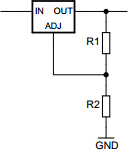# Linear regulator calculator

## Calculates properties of a linear regulator.

 Example 1: Must calculate the resistance of R2 to have output of 12 volts when R1 value is 220 ohms and regulator is LM317 View example Example 2: Must calculate the output voltage of a 3% tolerance regulator when R1 value is 120 ohms and R2 value is 680 ohms View example
 Output voltage volts Resistor R1 ohms Resistor R2 ohms Reference voltage volts ADJ-current amperes Tolerance %You may use one of the following SI prefix after a value: p=pico, n=nano, u=micro, m=milli, k=kilo, M=mega, G=giga

Fill in all values except the one you wish to calculate
• Output voltage - Output voltage of the regulator circuit
• Resistor R1 - Resistance of the resistor R1
• Resistor R2 - Resistance of the resistor R2
• Reference voltage - The internal reference voltage of the regulator
• ADJ-current - The internal adjustment pin current of the regulator (typically 50uA)
• Tolerance - The output voltage tolerance of the regulator